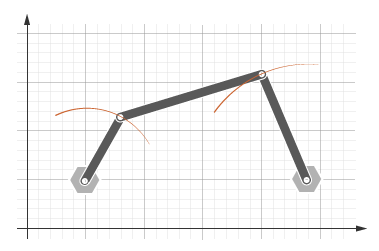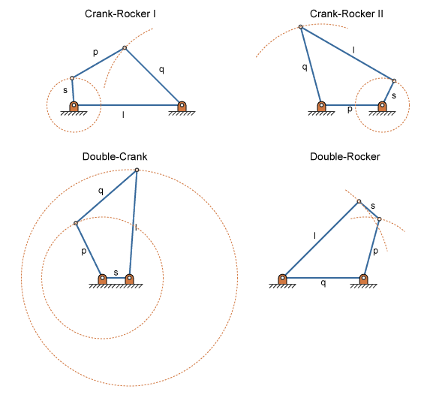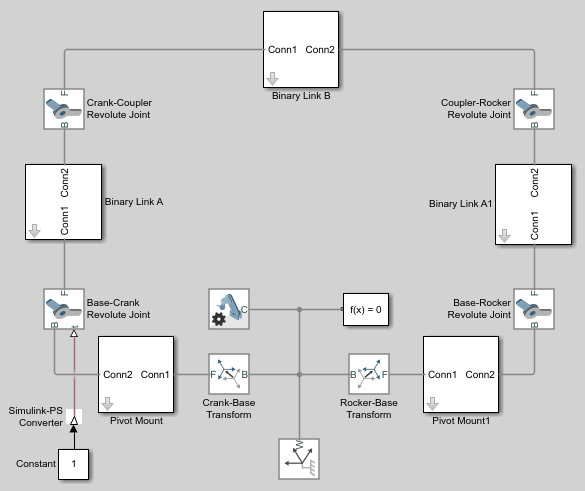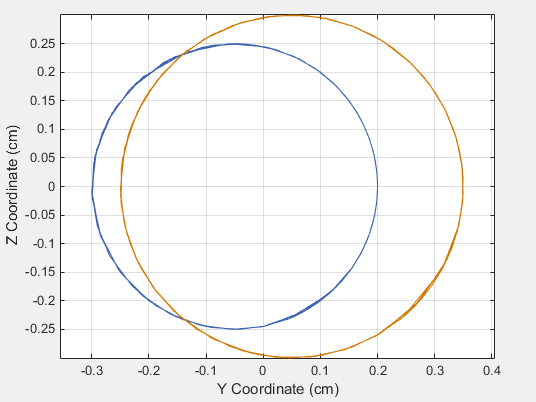## Specify Joint Actuation Torque

### Model Overview

In Simscape™ Multibody™, you actuate a joint directly using the joint block. Depending on the application, the joint actuation inputs can include force/torque or motion variables. In this example, you prescribe the actuation torque for a revolute joint in a four-bar linkage model.

Transform Sensor blocks add motion sensing to the model. You can plot the sensed variables and use the plots for kinematic analysis. In this example, you plot the coupler curves of three four-bar linkage types: crank-rocker, double-crank, and double-rocker.The four-bar linkage contains four links that interconnect with four revolute joints to form a planar closed loop. This linkage converts the motion of an input link into the motion of an output link. Depending on the relative lengths of the four links, a four-bar linkage can convert rotation into rotation, rotation into oscillation, or oscillation into oscillation.

Links go by different names according to their functions in the four-bar linkage. For example, coupler links transmit motion between crank and rocker links. The table summarizes the different link types that you may find in a four-bar linkage.

CrankRevolves with respect to the ground link
RockerOscillates with respect to the ground link
CouplerTransmits motion between crank and rocker links
GroundRigidly connects the four-bar linkage to the world or another subsystem

It is common for links to have complex shapes. This is especially true of the ground link, which may be simply the fixture holding the two pivot mounts that connect to the crank or rocker links. You can identify links with complex shapes as the rigid span between two adjacent revolute joints. In example Model a Closed-Loop Kinematic Chain, the rigid span between the two pivot mounts represents the ground link.

The type of motion conversion that a four-bar linkage provides depends on the types of links that it contains. For example, a four-bar linkage that contains two crank links converts rotation at the input link into rotation at the output link. This type of linkage is known as a double-crank linkage. Other link combinations provide different types of motion conversion. The table describes the different types of four-bar linkages that you can model.

Crank-rockerContinuous rotation-oscillation (and vice-versa)
Double-CrankContinuous rotation-continuous rotation
Double-rockerOscillation-oscillation

#### Grashof Condition

The Grashof theorem provides the basic condition that the four-bar linkage must satisfy so that at least one link completes a full revolution. According to this theorem, a four-bar linkage contains one or more crank links if the combined length of the shortest and longest links does not exceed the combined length of the two remaining links. Mathematically, the Grashof condition is:

 s+l ≤ p+q (1)
where:

• s is the shortest link

• l is the longest link

• p and q are the two remaining links

A Grashof linkage can be of three different types:

• Crank-rocker

• Double-crank

• Double-rocker

By changing the ground link, you can change the Grashof linkage type. For example, by assigning the crank link of a crank-rocker linkage as the ground link, you obtain a double-crank linkage. The figure shows the four linkages that you obtain by changing the ground link.### Modeling Approach

In this example, you perform two tasks. First you add a torque actuation input to the model. Then, you sense the motion of the crank and rocker links with respect to the World frame. The actuation input is a torque that you apply to the joint connecting the base to the crank link. Because you apply the torque at the joint, you can add this torque directly through the joint block. The block that you add the actuation input to is called Base-Crank Revolute Joint.

You add the actuation input to the joint block through a physical signal input port. This port is hidden by default. To display it, you must select `Provided by Input` from the Actuation > Torque drop-down list.

You can then specify the torque value using either Simscape or Simulink® blocks. If you use Simulink blocks, you must use the Simulink-PS Converter block. This block converts the Simulink signal into a physical signal that Simscape Multibody can use. For more information, see Actuating and Sensing with Physical Signals.

To sense crank and rocker link motion, you use the Transform Sensor block. With this block, you can sense motion between any two frames in a model. In this example, you use it to sense the [Y Z] coordinates of the crank and rocker links with respect to the World frame.

The physical signal output ports of the Transform Sensor blocks are hidden by default. To display them, you must select the appropriate motion outputs. Using the PS-Simulink Converter, you can convert the physical signal outputs into Simulink signals. You can then connect the resulting Simulink signals to other Simulink blocks.

In this example, you output the crank and rocker link coordinates to the workspace using Simulink To Workspace blocks. The output from these blocks provide the basis for phase plots showing the different link paths.

### Build Model

Provide the joint actuation input, specify the joint internal mechanics, and sense the position coordinates of the coupler link end frames.

#### Provide Joint Actuation Input

1. At the MATLAB® command prompt, enter `smdoc_four_bar`. A four bar model opens up. For instructions on how to create this model, see Model a Closed-Loop Kinematic Chain.

2. In the Base-Crank Revolute Joint block dialog box, in the Actuation > Torque drop-down list, select `Provided by Input`. The block exposes a physical signal input port, labeled t.

3. Drag these blocks into the model. The blocks enable you to specify the actuation torque signal.

LibraryBlock

4. Connect the blocks as shown in the figure. The new blocks are shaded gray.#### Specify Joint Internal Mechanics

Real joints dissipate energy due to damping. You can specify joint damping directly in the block dialog boxes. In each Revolute Joint block dialog box, under Internal Mechanics > Damping Coefficient, enter `5e-4` and press OK.

1. Add these blocks to the model. The blocks enable you to sense frame position during simulation.

LibraryBlockQuantity
Simscape > Multibody > Frames and TransformsTransform Sensor2
Simscape > Multibody > Frames and TransformsWorld Frame1

2. In the Transform Sensor block dialog boxes, select Translation > Y and Translation > Z. Resize the block as needed.

3. In the Output signal unit parameters of the PS-Simulink Converter block dialog boxes, enter `cm`.

4. In the Variable Name parameters of the To Workspace block dialog boxes, enter the variable names:

• `y_crank`

• `z_crank`

• `y_rocker`

• `z_rocker`

5. Connect and name the blocks as shown in the figure, rotating them as needed. Ensure that the To Workspace blocks with the z_crank and z_rocker variable names connect to the z frame ports of the Transform Sensor blocks. The new blocks are shaded yellow.### Simulate Model

Run the simulation. You can do this in the Simulink tool bar by clicking the run button. Mechanics Explorer plays a physics-based animation of the four bar assembly.Once the simulation ends, you can plot the position coordinates of the coupler link end frames, e.g., by entering the following code at the MATLAB command line:

```figure; plot(y_crank.data, z_crank.data, 'color', [60 100 175]/255); hold; plot(y_rocker.data, z_rocker.data, 'color', [210 120 0]/255); xlabel('Y Coordinate (cm)'); ylabel('Z Coordinate (cm)'); axis equal; grid on;```

The figure shows the plot that opens. This plot shows that the crank completes a full revolution, while the rocker completes a partial revolution, e.g., it oscillates. This behavior is characteristic of crank-rocker systems.#### Simulate Model in Double-Crank Mode

Try simulating the model in double-crank mode. You can change the four-bar linkage into a double-crank linkage by changing the binary link lengths according to the table.

BlockParameterValue
Binary Link ALength`25`
Binary Link BLength`20`
Binary Link A1Length`30`
Crank-Base TransformTranslation > Offset`5`
Rocker-Base TransformTranslation > Offset`5`

Update and simulate the model. The figure shows the updated visualization display in Mechanics Explorer.Plot the position coordinates of the coupler link end frames. At the MATLAB command line, enter:

```figure; plot(y_crank.data, z_crank.data, 'color', [60 100 175]/255); hold; plot(y_rocker.data, z_rocker.data, 'color', [210 120 0]/255); xlabel('Y Coordinate (cm)'); ylabel('Z Coordinate (cm)'); axis equal; grid on;```

The figure shows the plot that opens. This plot shows that both links complete a full revolution. This behavior is characteristic of double-crank linkages.